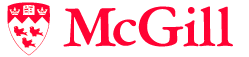# COMP/MATH 553: Algorithmic Game Theory

## Course Outline

• Introduction
• Games, solution concepts, auctions.

• Mechanism Design
• Social Choice Theory: Arrow's impossibility theorem, the Gibbard-Satterthwaite theorem;
• Second-price auction, sponsored search auction;
• Vickrey-Clarke-Groves mechanism, spectrum auctions;
• Myerson's Lemma: characterization of single-parameter incentive compatible mechanisms, revenue equivalence;
• Myerson's Optimal Auction;
• Prior-independent mechanisms: prophet inequality, Bulow-Klemperer;
• Multi-dimensional Mechanism Design;
• Stable Matching, Kidney Exchange

• Zero-sum Games
• Min-max theorem & Linear Programming Duality;
• Game Dynamics: fictitious play;
• No-regret Learning: multiplicative weights update method;
• Multi-player Zero-sum games.

• General Games
• Existence of Nash equilibria: proof via Sperner's lemma; emphasis on the combinatorial proofs of existence;
• Algorithms for computing Nash equilibria: the Lemke Howson algorithm, support enumeration algorithms, sampling methods, simplicial approximation algorithms;
• Complexity theory of total search problems and fixed points: definition of the complexity classes PLS, PPAD, PPP and relation to P, NP;
• The complexity of computing a Nash equilibrium: PPAD-completeness results;
• The complexity of pure Nash equilibria in congestion games: PLS-completeness results;
• Description method vs computational complexity in games; alternative game representations: graphical games, action graph games; emphasis on modeling the structure of the player interactions;
• Symmetries in games: algorithms for symmetric games; algorithms for anonymous games; relation to Central Limit Theorems in probability theory.

• Correlated Equilibria.
• Algorithms: Ellipsoid against hope;
• No internal-regret algorithms.

• Market Equilibria
• the Arrow-Debreu existence theorem;
• Algorithms for markets with linear utility functions;
• Tatonnement;
• PPAD-hardness of markets with Leontief utility functions.

• Price of Anarchy : selfish routing in networks, PoA in congestion games.